Courses

# SSC CPO Mock Test (GI & Reas) 5

## 50 Questions MCQ Test SSC CPO & Constable - Mock Tests & Previous Year Papers | SSC CPO Mock Test (GI & Reas) 5

Description
This mock test of SSC CPO Mock Test (GI & Reas) 5 for SSC helps you for every SSC entrance exam. This contains 50 Multiple Choice Questions for SSC SSC CPO Mock Test (GI & Reas) 5 (mcq) to study with solutions a complete question bank. The solved questions answers in this SSC CPO Mock Test (GI & Reas) 5 quiz give you a good mix of easy questions and tough questions. SSC students definitely take this SSC CPO Mock Test (GI & Reas) 5 exercise for a better result in the exam. You can find other SSC CPO Mock Test (GI & Reas) 5 extra questions, long questions & short questions for SSC on EduRev as well by searching above.
QUESTION: 1

Solution:
QUESTION: 2

Solution:
QUESTION: 3

### Choose the word which is least like the other words in the group .

Solution:
QUESTION: 4
Find out which word or name will be in the middle after the words or names are arranged in the alphabetical order.
Solution:
QUESTION: 5
Choose the number which is different from others.
Solution:
QUESTION: 6
Choose the group of letters which is different from others.
Solution:
QUESTION: 7
Complete the analogous pair.
Bleat : Bray :: Speak : ?
Solution:
QUESTION: 8
Complete the analogous pair.
Amber : Yellow : : Caramine : ?
Solution:
QUESTION: 9
Find the number of triangles in the given figure.
Solution:
QUESTION: 10
Complete the analogous pair.
Bizarre : Unusual :: Whimsical : ?
Solution:
QUESTION: 11
Complete the analogous pair.
Kannada : Karnataka :: ? Kerala
Solution:
QUESTION: 12
The post office is in the East of the school while my house is in the South of the school. The market is in the North of the post office. If the distance of the market from the post office is equal to the distance of my house from the school, in which direction is the market with respect to my house?
Solution:
QUESTION: 13

A man is facing north-west. He turns 90º in the clockwise direction, and then 135º in the anticlockwise direction. Which direction is he facing now ?

Solution: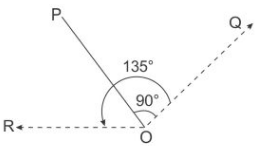The man initially faces in the direction OP. On moving 90 clockwise, the man faces in the OQ. On further moving 135º anticlockwise, he faces in the direction OR, which is West.

QUESTION: 14
If first 6 letter shall be written in opposite order, then the next 6 letter shall be written in opposite order and so on. If at the end Y will be interchanged by Z then which will be the fourth letter from left to the right of 13th letter?
Solution:
QUESTION: 15
Group the following figures into three classes on the basis of identical properties.
Solution: 1,5,7 have two similar elements, one inside the other.
2,4,8 have one element placed inside a different element.
3,6,9 have two similar elements, one inside the ohter and the area between the two elements is shaded.
QUESTION: 16

Find the missing character.

Solution:

The sequence in first column is ×5.Thus,1×5=5,5×5=25,25×5=125.
The sequence in third column is ×2.Thus¸7×2=14,14×2=28,28×2=56.
The sequence in second column is ×4.
∴∴Missing number = 12×4=48.

QUESTION: 17
Find the missing character.
Solution:
QUESTION: 18
Find the missing character.
Solution: In fig. (A), 93-(27+63)=3.
In fig. (B), 79-(38+37)=4.
$\therefore$In fig. (C), missing number =67-(16+42)=9.
QUESTION: 19
Choose the odd one out.
Solution:
QUESTION: 20
Choose the odd one out.
Solution:
QUESTION: 21
How many such letters are there in the word DECLARATION each of which is as far away from the beginning of the word as in the English alphabet?
Solution: Here we are looking for the distance of the word. For example C is at third position in english alphabets so if it at third position in the given word also, then it meets our requirement, else it does not.
So checking for each letter in the given word we see that D and E do not meet the requirement as they are occupying first and second position whereas their original positions are 4 and 5.
Checking in a similar manner, we can see that following words meet our requirement:
C,I
So there are only two words whose position in DECLARATION is same as their position in original english alphabets.
QUESTION: 22
The shaded area represents
Solution:
QUESTION: 23
If A = Athletes, B = Basketball players and C = Cricket fans, E = People above eight years of age and F = People below forty years of age, then the shaded area depicts.
Solution:
QUESTION: 24
If A stands for Addition , S stands for Subtraction, M stands for Multiplication, D stands for Division, G for Greater Than and L for Less than, Then which of the following alternatives will be logically correct.
Solution:
QUESTION: 25

select the correct combination of mathematical signs to replace * sign to balance the given equation.
24*3*2*12*3

Solution:
QUESTION: 26
If * means “is greater than”, @ means `is less then’; and \$ means “is equal to” and if a \$ b and b @ c, then
Solution: Replace the symbols with the meaning given against them. If a \$ b and b @ c would become a = b and b < c, then c > b or c > a ie, c * b, c * a are true from the given options.
QUESTION: 27
Complete the analogous pair.
Given Group : (5, 6, 22)
Solution:
QUESTION: 28

Choose the odd one out.

Solution:
QUESTION: 29
Choose the odd one out.
Solution:
QUESTION: 30

Choose the odd one out.

Solution:
QUESTION: 31

Choose the correct alternative that will continue the same pattern and fill in the blank.
5, 16, 49, 104, (....)

Solution:

The pattern is +11, +33, +55, .....
i.e. + (11 × 1), + (11 × 3), + (11 × 5), .....
i.e. 5 + (11 × 1) = 16
16 + (11 × 3) = 49
49 + (11 × 5) = 104
104 + (11 × 7) = 181

QUESTION: 32

Find the wrong number in the series.
16, 4, 2, 1.5, 1.75, 1.875,

Solution:

The series is ×0.25,×0.5,×0.75,×1,.......

QUESTION: 33
Choose the correct alternative that will continue the same pattern and fill in the blank.
121, 225, 361, (.....)
Solution:
QUESTION: 34
In this letter series, some of the letters are missing. Choose the correct letter given below -
c _ bbb _ _ abbbb _ abbb _
Solution:
QUESTION: 35
Choose the missing term out of the given alternatives.
U, O, I, ?, A
Solution:
QUESTION: 36
Choose the missing term out of the given alternatives.
C4X, F9U, I16R, ?
Solution:
QUESTION: 37
Below is given statement followed by two assumptions numbered I and II. You have to consider the statement and the following assumptions and decide which of the assumptions is implicit in the statement :
Statement : The Government has made an appeal to all the citizens to honestly pay income tax and file returns reflecting the true income level to help the Government to carry out developmental activities.
Assumptions:
I. People may now start paying taxes in response to the appeal.
II. The total income tax collection may considerably increase in the near future.
Solution:
QUESTION: 38
Below are two statements followed by three conclusions numbered I, II and III. You have to consider the statements and the following conclusions and decide which of the conclusion follows the statement.
Statements:
a. No room is tiger.
b. All tigers are goats.
Conclusions:
I. Some goats are rooms.
II. All goats are rooms.
III. Some goats are tigers.
Solution:
QUESTION: 39
If Sand is coded as Brick, Brick as House, House as Temple, Temple as Palace then where do you worship?
Solution:
QUESTION: 40
A bus for Bombay leaves every thirty minutes from a bus stand and enquiry clerk told a passenger that the bus had already left ten minutes ago and the next bus will leave at 9.35 a.m. At what time did the enquiry clerk give this information to the passenger?
Solution:
QUESTION: 41
In each of the following questions, four groups of three words each are given. Only one group in each does not share the underlying principle that combines the words. Find that group:
Solution:
QUESTION: 42
How many meaningful English words can be made with the letters ALEP using each letter only once in each word?
Solution: Reqd. words are : LEAP, PLEA, PALE and PEAL
QUESTION: 43

In the following questions, select a figure from amongst the four alternatives, which when placed in the blank space of fig. (X) would complete the pattern.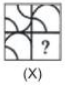Solution:
QUESTION: 44

These questions are based on code language which utilizes letters in the English Alphabet. In each question, there is a word written in capital letters, with one letter underlined. For each letter in that word there is a code written in small letters. That code is denoted by either (a), (b), (c), or (d), not in the same order. You have to find out the exact code for the underlined letter in the word. The number of the that code is the answer. Please note that the same letter appearing in other word(s) may be coded differently.

U E L

Solution:
QUESTION: 45

In each question below is given a group of letters followed by four combinations of digits/symbols marked (A), (B), (C) and (D). You have to find which of the combinations correctly represents the groups of letters based on the following coding system and the given conditions and mark the letter of that combination as the answer. If none of the four combinations correctly represents the group of letters, mark (E) i.e. 'None of these' as the answer.
Letter :
A M P J E I R T W B U K Y Z H
Digit/Symbol Code:
1 9 2 @ 3 © 4 8 7 \$ 6 % 5 * δ
Conditions :
(i) If the first letter is consonant and the last letter is a vowel, their codes are to be interchanged.
(ii) If the first letter is vowel and the last letter is consonant, both are to be coded as the code for the vowel.

UPAMYE

Solution:
QUESTION: 46

In each question below is given a group of letters followed by four combinations of digits/symbols marked (A), (B), (C) and (D). You have to find which of the combinations correctly represents the groups of letters based on the following coding system and the given conditions and mark the letter of that combination as the answer. If none of the four combinations correctly represents the group of letters, mark (E) i.e. 'None of these' as the answer.
Letter :
A M P J E I R T W B U K Y Z H
Digit/Symbol Code:
1 9 2 @ 3 © 4 8 7 \$ 6 % 5 * δ
Conditions :
(i) If the first letter is consonant and the last letter is a vowel, their codes are to be interchanged.
(ii) If the first letter is vowel and the last letter is consonant, both are to be coded as the code for the vowel.

JPERTB

Solution:
QUESTION: 47

Select the correct mirror-image of the Figure (X) from amongst the given alternatives.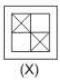Solution:
QUESTION: 48

Find out from amongst the four alternatives as to how the pattern would appear when the transparent sheet is folded at the dotted line.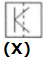Solution:
QUESTION: 49

Each of the following question consist of five problem figure marked 1, 2, 3, 4, 5 followed by Answer figure A, B, C, D and E. Select of figure from the answer figure which will continue the same series as given in the problem figure.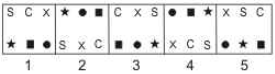Solution:
QUESTION: 50

In the questions, figure (X) is embedded in one of following figures. Find out the correct alternative.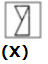Solution: